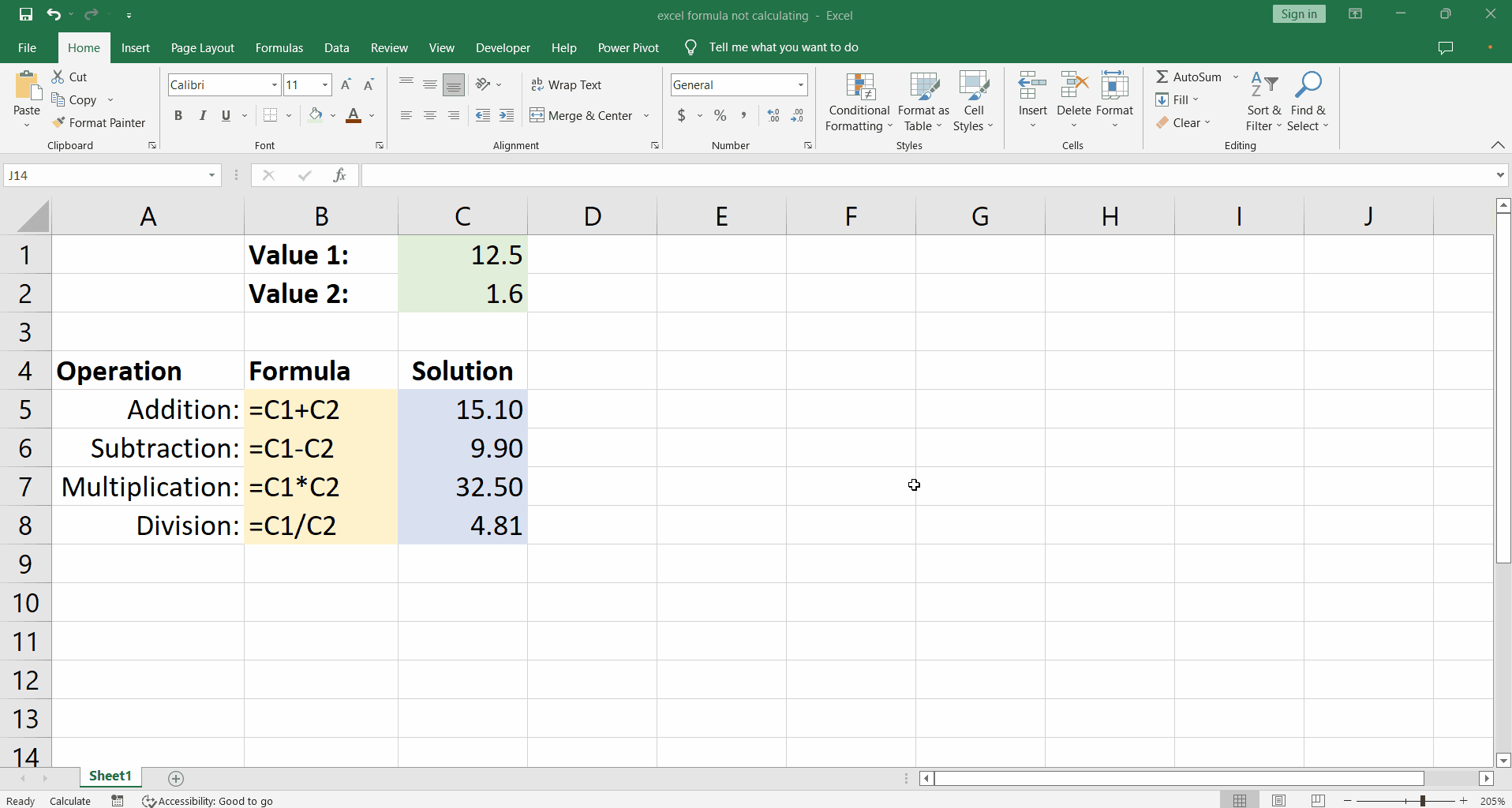# How to fix excel formula is not calculating upon changing values issue

Suppose we have a sheet with different formulas implemented for a pair of values in the sheet such as the arithmetic operations i.e. addition, subtraction, division and multiplication. The dataset is shown below.Sometimes you might encounter a workbook where you have modified a formula or a calculation but the worksheet does not appear to reflect the change. This is a problem with excel calculations.

### Step 1 – Confirming the issue– Let’s change one of the input values, say we change Value 2 to 1.6 instead of 2.6.

– However, there is no change in the Solution to any of the formulas that we have applied.

### Step 2 – Solving the problem– In order to solve the problem go to the Calculation group on the Formulas tab find the Calculation Options drop down and click Automatic.

– You will see that the values are immediately updated as excel performs calculations right away.